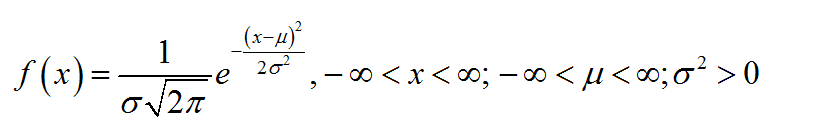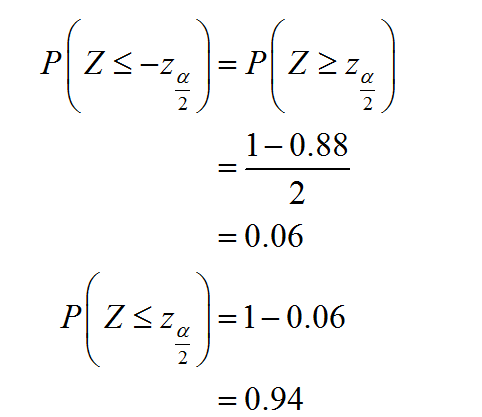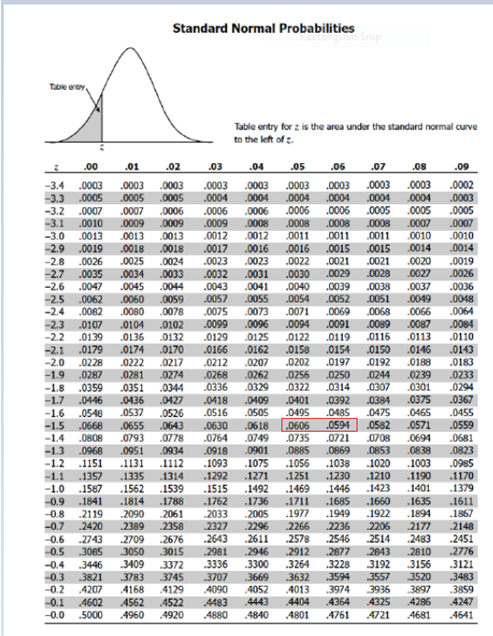# Find zα/2 for each of the following confidence levels used in estimating the population mean. (You may find it useful to reference the z table. Round your answers to 3 decimal places.)88%=

Question
103 views

Find zα/2 for each of the following confidence levels used in estimating the population mean. (You may find it useful to reference the z tableRound your answers to 3 decimal places.)

88%=

check_circle

Step 1

The normal distribution:

A continuous random variable X is said to follow normal distribution with mean µ and standard deviation σ if the probability density function of X is,Step 2

Calculating the z-value:

The required z-value is calculated as follows:Step 3

The standard normal table is given below:From the above table, it is clear that 0.60 is the average of the values 0.0606 and 0.0594. There for –Zα/2 is –1.555 (= (-1.55+-1.56)/2)

...

### Want to see the full answer?

See Solution

#### Want to see this answer and more?

Solutions are written by subject experts who are available 24/7. Questions are typically answered within 1 hour.*

See Solution
*Response times may vary by subject and question.
Tagged in